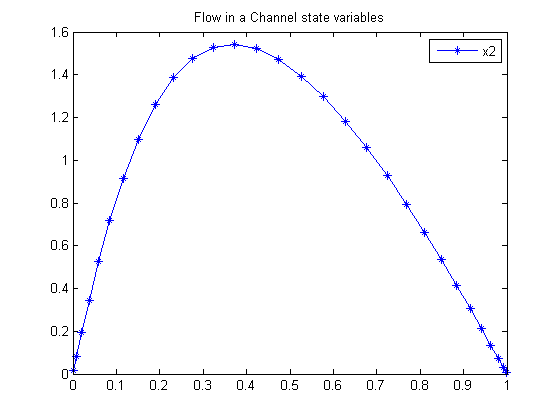24  Flow in a Channel

Benchmarking Optimization Software with COPS Elizabeth D. Dolan and Jorge J. More ARGONNE NATIONAL LABORATORY

24.1  Problem Formulation

Find u(t) over t in [0; 1 ] to minimize

 J = 0

subject to:

 d4u dt4
= R*(
 du dt
*
 d2u dt2
u*
 d3u dt3

 u0 = 0
 u1 = 1
 du dt
0 = 0
 du dt
1 = 0
 R = 10

After some transformation we get this problem:

 dx1 dt
= x2
 dx2 dt
= x3
 dx3 dt
= x4
 dx4 dt
= R*(x2*x3x1*x4
 x1(0) = 0
 x1(1) = 1
 x2(0) = 0
 x2(1) = 0

Reference: 

24.2  Problem setup

toms t
p = tomPhase('p', t, 0, 1, 30);
setPhase(p);

tomStates x1 x2 x3 x4

x0 = icollocate({x1 == 3*t.^2 - 2*t.^3
x2 == 2*t - 6*t.^2
x3 == t - 12*t
x4 == -12});

% Boundary constraints
cbnd = {initial({x1 == 0; x2 == 0})
final({x1 == 1; x2 == 0})};

% Various constants and expressions
R = 10;

% ODEs and path constraints
ceq = collocate({dot(x1) == x2
dot(x2) == x3; dot(x3) == x4
dot(x4) == R*(x2.*x3-x1.*x4)});

% Objective
objective = 1; %(feasibility problem)

24.3  Solve the problem

options = struct;
options.name = 'Flow in a Channel Steering';
solution = ezsolve(objective, {cbnd, ceq}, x0, options);

% Extract optimal states and controls from solution
t  = subs(collocate(t),solution);
x1 = subs(collocate(x1),solution);
x2 = subs(collocate(x2),solution);
x3 = subs(collocate(x3),solution);
x4 = subs(collocate(x4),solution);
Problem type appears to be: lpcon
Starting numeric solver
===== * * * =================================================================== * * *
TOMLAB - Tomlab Optimization Inc. Development license  999001. Valid to 2011-02-05
=====================================================================================
Problem: ---  1: Flow in a Channel Steering     f_k       1.000000000000000000
sum(|constr|)      0.000000000018584877
f(x_k) + sum(|constr|)      1.000000000018584900
f(x_0)      0.000000000000000000

Solver: snopt.  EXIT=0.  INFORM=1.
SNOPT 7.2-5 NLP code
Optimality conditions satisfied

FuncEv    1 ConstrEv   11 ConJacEv   11 Iter    9 MinorIter   91
CPU time: 0.062500 sec. Elapsed time: 0.078000 sec.

24.4  Plot result

figure(1)
plot(t,x2,'*-');
legend('x2');
title('Flow in a Channel state variables');